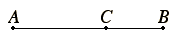# Definition 3

A straight line is said to have been cut in extreme and mean ratio when, as the whole line is to the greater segment, so is the greater to the less.

## Guide

 The line AB is cut in extreme and mean ratio at C since AB : AC = AC : CB.A construction to cut a line in this manner first appeared in Book II, proposition II.11. Of course that was before ratios were defined, and there an equivalent condition was stated in terms of rectangles, namely, that the square on AC equal the rectangle AB by BC. That construction was later used in Book IV in order to construct regular pentagons and 15-sided polygons (propositions IV.10 through 12 and 16). Now that the theory of ratios and proportions has been developed, it is time to define this section as a ratio, rather than using rectangles. An alternate construction is given in proposition VI.30. Yet one more characterization of cutting a line in extreme and mean ratio appears in Book XIII proposition XIII.1: the square on the greater segment added to the half of the whole is five times the square on the half. That property is used to analyze the sides of the icosahedron and dodecahedron constructed in propositions XIII.16 and XIII.17